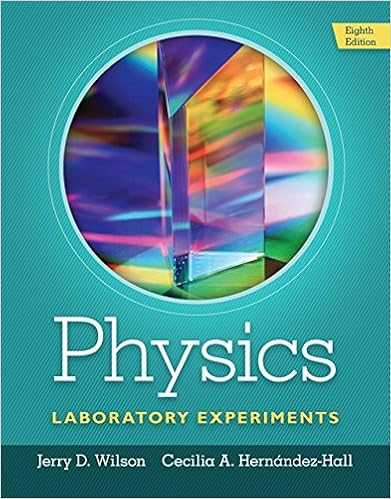# Law of sine to find its direction in one instance the

• Lab Report
• 5
• 83% (6) 5 out of 6 people found this document helpful

This preview shows page 2 - 3 out of 5 pages.

##### We have textbook solutions for you!
The document you are viewing contains questions related to this textbook.The document you are viewing contains questions related to this textbook.
Chapter 6 / Exercise 8
Physics Laboratory Experiments
WilsonExpert Verified
law of sine to find its direction in one instance. The components method will also be used. Theory A vector is a physical quantity that has both magnitude and direction. Examples include force, weight, displacement, velocity and acceleration. On the other hand, a scalar is a physical quantity that has magnitude only, examples speed, mass, distance, temperature, population. A vector may be represented by a letter example with a bar on top indicating the direction, or by a bold letter, example A . A vector A can be written as a sum of two vectors A x and A y along the x and the y-axis respectively, as shown . A x and A y are called the components of vector A and are given by A x = A cosα A y = A sinα α (≤ 90 0 ) is the angle vector A makes with the x-axis. In order to find the resultant vector R of a system of vectors A , B , C , etc we follow these steps: a) Find the x and y components for each vector using the above equations. i.e find A x, B x, C x ... and A y, B y, .... : C y
##### We have textbook solutions for you!
The document you are viewing contains questions related to this textbook.The document you are viewing contains questions related to this textbook.
Chapter 6 / Exercise 8
Physics Laboratory Experiments
WilsonExpert Verified
•••